## Introduction

In the recent years, a considerable effort has been made in the development of polymer-based micro- and nano-fabrication techniques for applications in micro-electro-mechanical systems (MEMS). Techniques such as electron beam lithography (EBL) and atomic force microscopy lithography (AFML) have proven successful in transferring nano-patterns with line width of the order of few tenths of nanometers. However, the cost and the deficient processability for mass production have caused the emergence of promising techniques that can retain or improve the resolution of EBL and AFML and circumvent their inherent shortcomings. One of these techniques is thermal nano-imprint lithography (henceforth simply referred to as nano-imprint lithography or NIL)  offering resolutions below 10 nm.

Nano-imprint lithography (NIL) is essentially a two-step process. In the first step, a resist film (a thermoplastic polymer) is cast onto a hard substrate and heated above its glass transition temperature. A rigid stamp (or mold) containing some well-defined structures patterned on its surface is brought into contact with the film and pressed in so that the negative replica of the stamp will be transferred. The pressure load is held for a period of time and then the stamp is cooled down until its temperature is below the glass transition temperature of the polymer. This ensures the mechanical stability of the film and then demolding can be carried out.

The NIL operational window  covers pressures up to 200 bars, temperatures of about 200 °C (it has been experimentally found that operation temperatures of 70–90 °C above the glass transition temperature of the polymer are suitable for the imprint), and stamp sizes of 2 cm × 2 cm. The fact that the patterning can be done over large areas is particularly attractive in connection with the manufacturing of high-volume data storage and high-speed data-processing components.

Nano-imprint lithography (NIL) can be regarded as a particular case of hot embossing lithography (HEL), but with the specific characteristic that the magnitude of the thickness of the samples is comparable to the height of the indents in the mould. Hence, the patterning is not a surface modulation process, as the HEL, but demands the transport of a large amount of the polymer from areas in contact with the stamp into the cavities. In most cases, NIL is an isothermal process where both stamp and sample have identical temperatures.

Many related NIL publications have appeared in the last decade. Only few of them have consistently addressed the investigation of polymer flow during imprint. Different experimental approaches have shown that the flow mechanism of polymers into nano-structures [3, 4] is not trivial, and this has been attributed to a variety of causes such as the type of flow, the stamp geometry, the viscoelastic properties of the polymer, as well as the role played by surface tension effects. The latter includes attraction forces of different nature between the stamp and the polymer [5, 6].

The experimental work has often been followed by attempts to simulate the flow during imprint mainly for the cases of periodic squared arrays or squared cavities. Simulations have been carried out using finite element methods, and all the flow problems were solved in a two-dimensional framework. Many of the numerical investigations adopted a general scope focusing in the understanding of the flow into micro/submicrometer structures.

Most of the early approaches treated the polymer either as a purely viscous fluid [7, 8] (sometimes using generalized Newtonian models) or as purely elastic materials . In a few cases, the surface tension of the polymer was taken into consideration but interfacial tension between polymer and stamp was not accounted for. To our knowledge, only two approaches considered viscoelasticity in the material. Hirai et al.  modeled the polymer flow using a linear viscoelastic constitutive equation and established a qualitative comparison with their experiments. The only study with relevance to the NIL or HEL process, applying a nonlinear constitutive equation is that of Eriksson et al. . Eriksson et al.  addressed both experimentally and numerically a compression molding on the millimeter scale combined with the filling of periodic squared arrays at micrometer length scales. Probably due to the applied nonlinear constitutive equation, they obtained a quantitative agreement between the filling profiles measured in the experiments and those provided by numerical simulations. The latter approach used an integral constitutive equation of the K-BKZ type [12, 13], within a Molecular Stress Function (MSF) approach , to describe the dynamics of the polymer.

## Polymer Melt as a Structured Liquid

The physics in the NIL is the isothermal flow of polymer melts. Polymers are structured systems where order phenomena develops the stresses in the material during flow (Pierre-Gilles de Gennes ). The reptation theory by Doi and Edwards  was the first contribution toward an exact formulation for the flow of polymers. Since then progress has been scarce. The ‘interchain pressure’ concept by Marrucci and Ianniruberto  is currently the only theoretical approach capable of accurately predicting the published homogeneous flow data for molar mass distributed as well as structurally well-defined polymer melts . Just recently, Wagner et al. [22, 23] and Rasmussen et al.  have suggested models capable of modeling the flow of polymer melts in general. These models are based on the ‘interchain pressure’ concept by Marrucci and Ianniruberto , and a Molecular Stress Function constitutive model . The latter is a generalization of the ideas and model of Doi and Edwards .

The general form of MSF constitutive model is written as a memory-weighted time integral over a strain tensor and the square of the molecular stress function,f;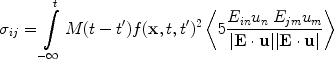(1)

Here,M(tt′) is the linear viscoelastic memory function and the strain,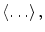is the independent alignment tensor from the Doi–Edwards reptation theory. The theoretical basis is the idea of a tube segment of unit length and orientation given by the unit vector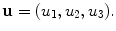In the stress-free state, u is deformed into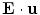in the current state. The components of the macroscopic displacement gradient tensor, E, are given by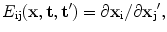i = 1, 2, 3 and j = 1, 2, 3.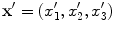are the coordinates of a given particle in the stress-free reference state (time t′), displaced to coordinates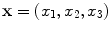in the current state (time t). The angular brackets,denote an average over a unit sphereThe variables in the displacement gradient tensor, x and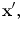are indexed by a Cartesian coordinate system attached to the particle. This corresponds to the use of Lagrangian variables.

Please notice, the flow physics depends on the choice of the molecular stress function,f[22, 24].

## Flow Modeling of Polymer Melts in NIL

The modeling of the NIL process requires time dependency in the applied method. A scant effort has been made in the development of numerical methods capable of handling time-dependent flow of integral constitutive equations . To perform a realistic modeling, it will (at least) require a fully three dimensional as well as free surface viscoelastic flow. The method of Wapperom  and the one by Rasmussen  both apply Lagrangian particle variables. Therefore, both methods can handle (large) movement of moving surfaces or interfaces without extra effort. The movement can be free or specified. Only the approach by Rasmussen  has been numerically formulated in three-dimensional (3D), although the step from 2D to 3D in most cases is a minor problem.

The major concern in fully three-dimensional computations is the immense increase in the number of unknown variables. Three-dimensional time-dependent computations in most cases require an efficient numerical formulation as well as code parallelization. Therefore, we will use the recent method by Marín et al. . This method is a Lagrangian finite element method, based on a proven convergent Galerkins principle . In a Lagrangian method, the node point follows the particle movement. The particle variables and the pressure field are approximated by quadratic (tetrahedral) and linear interpolation functions, respectively. The method is third-order accurate in both space and time, and is numerically stable for all chosen time steps sizes. The equations are solved by a robust Newton-Raphson iterative scheme and the code is fully parallelized.

The use of (Lagrangian) variables indexed by a coordinate system attached to the particle enables the numerical method to handle arbitrary large movement of the material freely moving surfaces or interfaces. The modeling of a NIL, as well as any other mold filling problem, is complicated by the presence of dynamic movement of the contact line between the melt and stamp during filling. It is therefore essential to apply an easy handling of this. Here, we treat the contact of the particles as follows: the time and position of the contact to a solid surface of the particles in nodal points (in the finite element discretization) was calculated with an explicit second order prediction. Any particle (e.g., node point) is then attached (e.g., sticking) to the solid mold, starting from the time (and position) of contact.

The finite element flow solver used here has been adapted to simulate the filling of an arbitrary stamp morphology. We will consider the example depicted in Fig. 1 where a stamp containing a surface patterned with periodic squared sinus cavities aligned with each other and equally spaced is pressed against a polymeric film of thickness T. Exploiting the symmetry, the simulation domain can be reduced to the region delimited by the blue line.

For simplicity, we will only use a molecular stress function f = 1. The modeling is then based on a liquid described constitutively by the independent alignment strain tensor from the Doi–Edwards reptation theory only. This corresponds to the exact flow properties of polymers melts when the ‘Interchain Pressure’ is relaxed. Currently, a relation between the ‘Interchain Pressure’ relaxation and the linear viscoelastic relaxation has not been established, although the ‘Interchain Pressure’ seems to relax at time scale in the size of the Rouse time. Further, we have applied a memory function as a continuous BSW spectrum  given by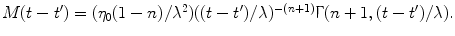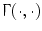is the incomplete gamma function, η0 the zero-shear viscosity and λ the maximal relaxation time constant. The BSW represent the linear viscoelastic dynamic of a monodisperse melt and is easily extended to broadly distributed polymers. In all computations, we use a Currie  approximation of the independent alignment tensor from the Doi–Edwards reptation theory.

A non-dimensionalization of the the stress (e.g., equation of motion), using non-dimensional variables as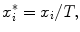t* = t/λ and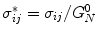, can be applied.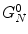is the elastic plateau modulus for the polymer and T the initial thickness of the substrate. Introducing these non-dimensional variables, the mass conservation as well as the momentum equations will only contain one non-dimensional parameter,n. We have used a fixed value of n = 0.07 here.

The initial unstructured finite element mesh is shown in Fig. 2(top and left) along with the shape of the cavity (denoted by the solid line). Non-slip boundary conditions are specified on the bottom plane of the domain. The three lateral planes are treated as symmetry planes. The top plane contains two differentiated regions; the semicircular one that is treated as a free surface and the secondary region formed by the nodes initially in contact with the mold. The flow can be induced either moving the initial contact area at a given velocity or applying a pressure load on it. Here, we have applied a constant velocity.